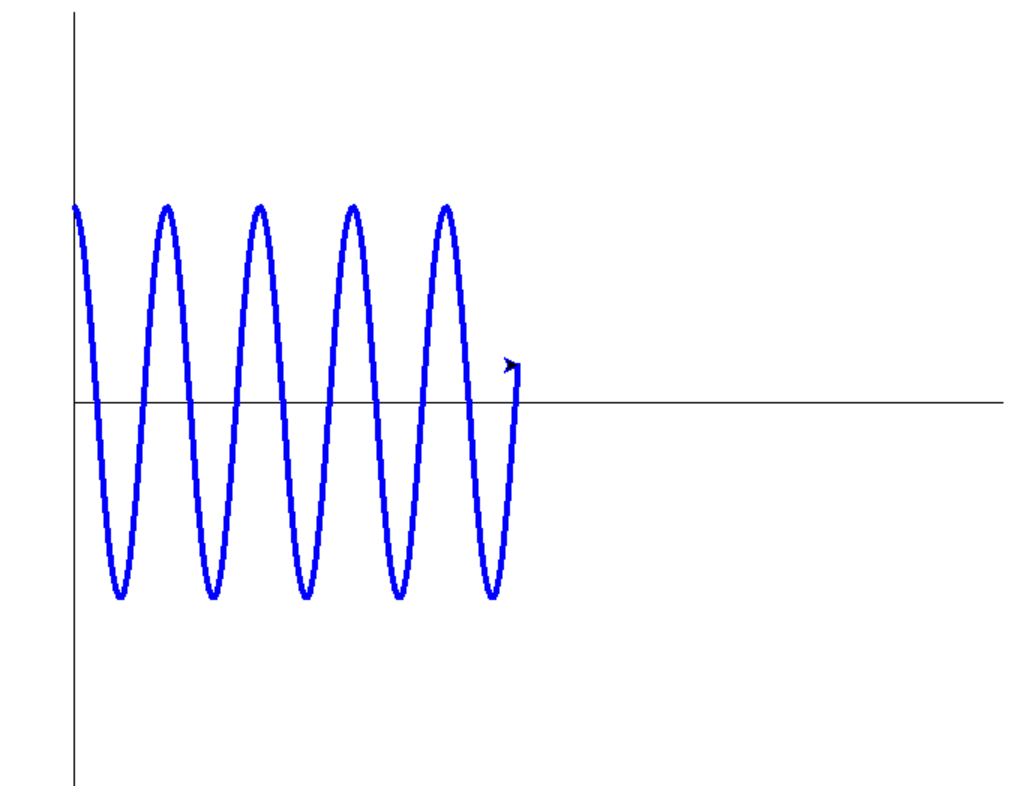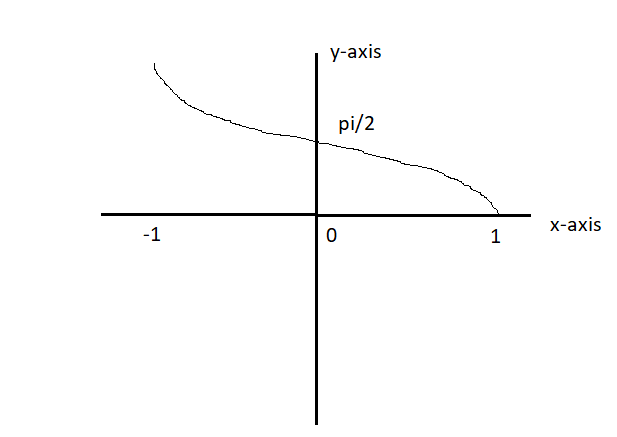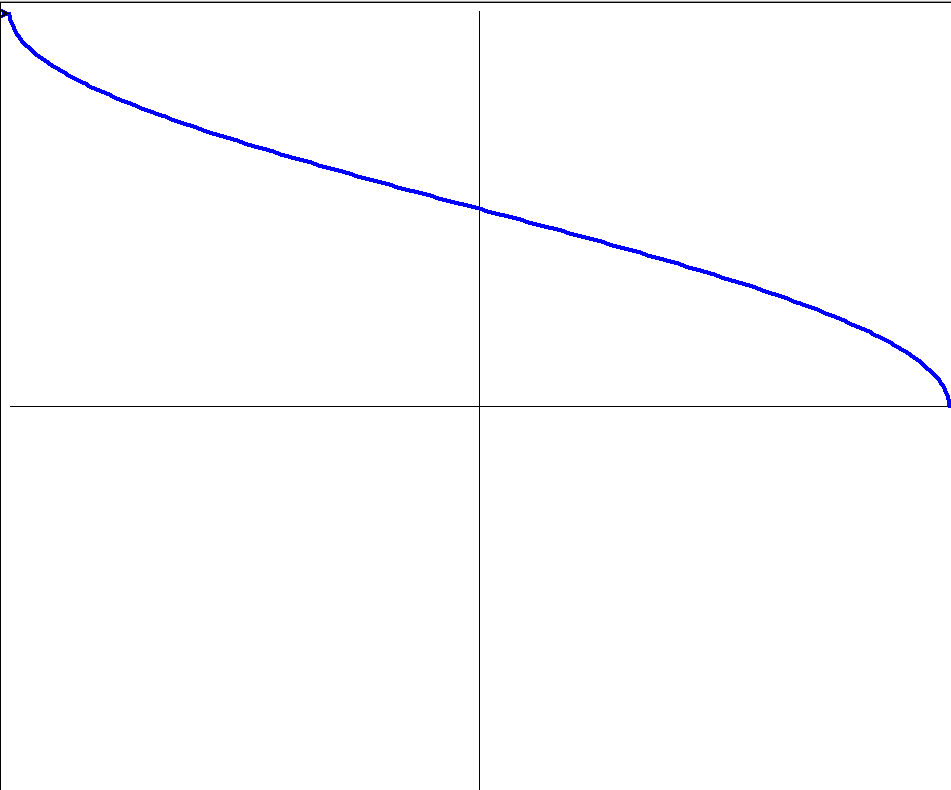# How to make a cosine wave graph in Python turtle?

• Last Updated : 19 Aug, 2022

In this article, we will learn how to draw a Cosine wave and the inverse of a cosine wave using a turtle in Python.

## What is Cosine?

The Cosine function, also written as cos or cos(x), reduces the hypotenuse of a right triangle to the projection onto the x-axis. cosine signal waveform with a shape identical to that of a sine wave it’s occurring exactly before one by four(1/4) cycle of the sine wave.

`Cos θ = Adjacent side/Hypotenuse`

The Cosine graph, and their degree

Cos Waveform:Example 1: Generating Cosine wave

In this example, we will import the required module and set the coordination, after that we will draw vertical and horizontal lines to draw our cosine wave.

## Python3

 `import` `math``import` `turtle` `win ``=` `turtle.Screen()``win.bgcolor(``"white"``)` `# coordinate setting``win.setworldcoordinates(``0``, ``-``2``, ``3600``, ``2``)``t ``=` `turtle.Turtle()` `# Draw a vertical line``t.goto(``0``, ``2``)``t.goto(``0``, ``-``2``)``t.goto(``0``, ``0``)` `# Draw a Horizontal line``t.goto(``3600``, ``0``)``t.penup()``t.goto(``0``, ``1``)``t.pendown()` `t.pencolor(``"blue"``)``t.pensize(``4``)` `# Generate wave form``for` `x ``in` `range``(``3600``):``    ``y ``=` `math.cos(math.radians(x))``    ``t.goto(x, y)`

Output:## What is Inverse Cosine Wave?

Inverse cosine is also known as arccosine. It is reciprocal of the Cosine wave. Cosine inverse of the same ratio will give the measure of the angle, y= cos -1(x) <=> cos y = x. Here, the cosine function is equal to the Adjacent side divided by the hypotenuse, and Each range value between -1 to 1 is within the limited domain (0,180).

`θ = Cos -1(Adjacent side/hypotenuse)`

The Inverse Cosine graph, and their degree:

Cos inverse Waveform:Example 2: Inverse Cosine

In this example, we will import the required module and set the coordination, after that we will draw vertical and horizontal lines to draw our Inverse cosine wave.

## Python3

 `import` `math``import` `turtle``win ``=` `turtle.Screen()``win.bgcolor(``"white"``)` `# coordinate setting``win.setworldcoordinates(``-``1``, ``-``180``, ``1``, ``180``)``t ``=` `turtle.Turtle()` `# Draw a Horizontal line``t.goto(``1``, ``0``)``t.goto(``-``1``, ``0``)``t.penup()``t.goto(``0``, ``0``)``t.pendown()` `# Draw a vertical line``t.goto(``0``, ``180``)``t.goto(``0``, ``-``180``)``t.penup()``t.goto(``1``, ``0``)``t.pendown()``t.pencolor(``"blue"``)``t.pensize(``4``)` `# Generate wave form``for` `y ``in` `range``(``0``, ``180``):``    ``x ``=` `math.cos(math.radians(y))``    ``t.goto(x, y)`

Output:My Personal Notes arrow_drop_up